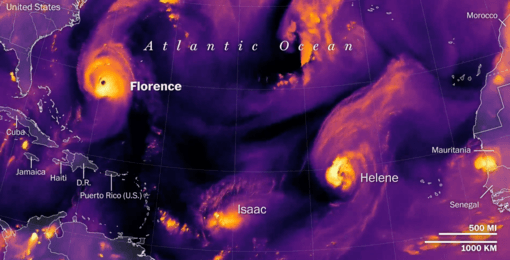# Some Dimensions of Hurricane FlorenceThe estimates which follow are based on rough quantitative data about Hurricane Florence, which were reported on 12 September 2018 by several news outlets.

1) Maximum sustained winds (morning, 12 September 2018):

215 km/hr = 134 mph.

2) Diameter (width) of the eye:

~40 km = ~25 miles.

3) Diameter (width) of the storm:

~660 km = ~410 miles.

4a) Area of the storm:

~238,000 km^2 = ~91,820 square miles.

4b) Area of storm calculated (by MG,Jr.) based on reported diameter:

342,000 km^2 = 132,000 square miles.

4c) Diameter of storm calculated (by MG,Jr.) based on reported area: 550.4 km = 342 miles

Other dimensional features, reported on 12 September 2018, are:

5) Largest ocean waves (which occur in the northeast quadrant of the storm): 83 feet = 25 meters.

6) Peak wind speed dropped (by afternoon 12 September 2018) to: 125 mph = 201 km/hr.

7) Size of the (inner high-speed) windfield expanded to a diameter

(the width, with eye in center) of:

140 miles = 226 km.

8) The hurricane is expected to bring a storm surge (onto the coast) upwards of 6 feet (1.8 m) in parts of the coastline, including up to 13 feet (4 m) from Cape Fear north to Cape Lookout.

9) The expected rainfall is up to 40 inches (1 m) in isolated areas in the Carolinas and anywhere between 6 to 12 inches (15 to 30 cm) elsewhere in the Appalachians and Mid-Atlantic region.

10) Expected cost of storm damages: \$170 billion; which would make Hurricane Florence the most expensive hurricane to hit the continental U.S.

Using an average height of 16 km (10 miles) for hurricanes:

11) The volume of the (inner, high speed) windfield is estimated to be: ~642,000 km^3 = ~154,000 cubic miles.

Using an average diameter (width) of 600 km = ~372 miles, for Hurricane Florence:

12) The area of the storm is:

~282,700 km^2 = ~109,100 square miles.

13) The volume of the entire storm is estimated to be:

~4,524,000 cubic km = ~1,084,000 cubic miles.

Now, a more speculative calculation, of RAINFALL (by MG, Jr.):

Assuming:

a) storm area = 282,700 square kilometers = ~109,100 square miles,

b) storm height = 16 km = ~10 miles,

c) storm volume = 4,524,000 cubic km = ~1,084,000 cubic miles,

and

d) 96.5% of standard air density (which is 1.204 kg/m^3 at sea level)

in the storm volume, to account for the “low pressure” of the system, which is primarily at the center, and rising toward the edge; so an average air density of 1.162 kg/m^3 for the storm,

e) 90% relative humidity at 70 degrees Fahrenheit, everywhere (an averaging), then, the water content in the storm is: 100 grains of water (0.0143 lb) per lb of dry air.

Using liquid water density at 1 gram/cc = 1 kg/1000cc = 1000kg/m^3, the amount of water (humidity) per VOLUME (of atmospheric space in the storm) is:

0.036633 lb of water per cubic meter =

0.0166166 kg of water per cubic meter =

16,616,600 kg of water per cubic kilometer (of air space in the storm).

Thus, the total mass of liquid water in the storm (of volume 4,524,000 cubic km) is: 75,173,498,400,000 kg of liquid water (75.173… trillion kg = 75.173… thousand-billion kilograms).

The volume of liquid water ALONE (for the entire storm) is: 75.1734984 km^3 (75.173… cubic kilometers).

For the area of this storm (282,700 km^2), the depth of rainfall

(if all the water rains out over the entire storm area) is: 0.266 meters = 26.6 centimeters = 10.5 inches.

Rain over real terrain will collect into flood streams of greater depth (for lower lying areas).

Also, the rainfall near the center of the hurricane will be much higher (a 40 inch = 1 meter expectation was reported);

The rainfall closer to the edge of the storm, and everywhere late in its lifetime, will be lighter (a 6-to12 inch = 15-to-30 centimeter expectation was reported).

So, my estimate of 10-11 inches of rainfall, averaged for the entire storm area (282,700 square kilometers = ~109,100 square miles), seems reasonable.

Source (1 to 4):

https://globalnews.ca/news/4443132/how-big-is-hurricane-florence/

Source (5 to 10):